2018
02-22

# 函数式编程简介

### 例子一 累加运算

``````// sum
List<Integer> nums = Arrays.asList(0, 1, 2, 3, 4, 5, 6, 7, 8, 10);

public static Integer sum(List<Integer> nums){
int result = 0;
for (Integer num : nums) {
result += num;
}

return result;
}

sum(nums); // -> 46
``````

``````Arrays.asList(0, 1, 2, 3, 4, 5, 6, 7, 8, 10).stream().reduce(0, Integer::sum);
``````

``````(apply + [0 1 2 3 4 5 6 7 8 10]) ; -> 46
#_(reduce + [0 1 2 3 4 5 6 7 8 10])
``````

### 例子二 fabonacci数列

``````// java
public static int fibonacci(int number){
if (number == 1) {
return 1;
}
if(number == 2) {
return 2;
}

int a = 1;
int b = 2;
for(int cnt = 3; cnt <= number; cnt++) {
int c = a + b;
a = b;
b = c;
}
return b;
}
``````
``````// java8
Stream.iterate(new int[]{1, 1}, s -> new int[]{s, s + s})
.limit(10)
.map(n -> n)
.collect(toList())
// -> [1, 2, 3, 5, 8, 13, 21, 34, 55, 89]
``````
``````// clojure
(->> (iterate (fn [[a b]] [b (+ a b)]) [1 1])
(map second)
(take 10))
; -> (1 2 3 5 8 13 21 34 55 89)
``````

## 函数式编程的历史

### 从Hilbert 23个数学难题谈起

1900年，Hilbert 提出了数学界悬而未决的10大问题，后续陆续添加成了23个问题，被称为著名的 Hilbert 23 Problem。针对其中第2个决定数学基础的问题——算术公理之相容性，年轻的哥德尔提出了哥德尔不完备定理，解决了这个问题形式化之后的前两点，即数学是完备的吗？数学是相容的吗？哥德尔用两条定理给出了否定的回答。所谓不完备，即系统中存在一个为真，但是无法在系统中推导出来的命题。比如：U说：“U在PM中不可证”。虽然和说谎者很类似，但其实有明显的差异。我们可以假设U为可证，那么可以推出PM是矛盾（不相容）的；但是假设U不可证，却推导不出PM是矛盾的。U的含义是在M中不可证，而事实上，它被证明不可证，所以U是PM中不可证的真命题。基于第一条不完备定理，又可以推导出第二条定理。如果一个（强度足以证明基本算术公理的）公理系统可以用来证明它自身的相容性，那么它是不相容的。

### Lambda Calculus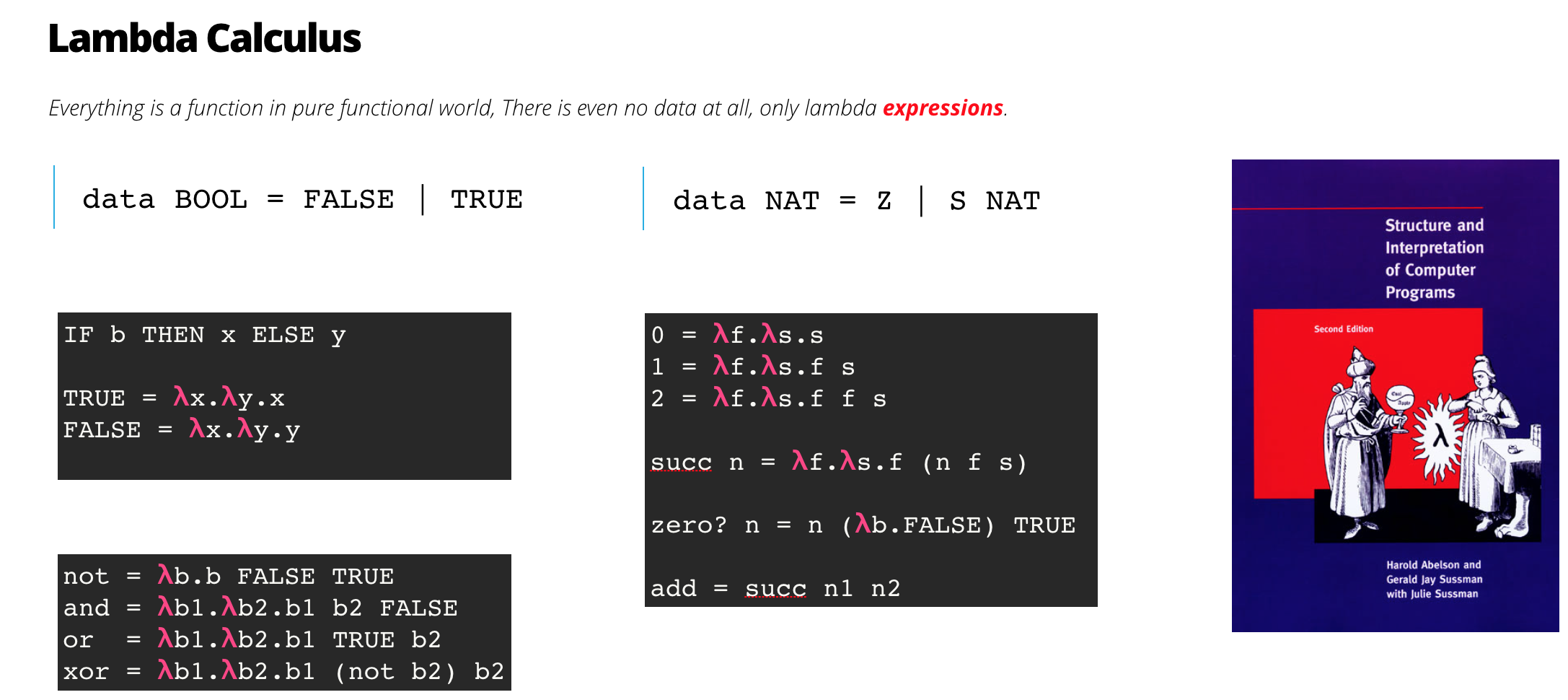Lambda 表达式包含三个要素

1. 变量
2. lambda 抽象
3. lambda 应用
据此我们可以用函数给出布尔值的定义
``````data BOOL = FALSE | TRUE
TRUE = λx.λy.x
FALSE = λx.λy.y

not = λb.b FALSE TRUE
and = λb1.λb2.b1 b2 FALSE
or  = λb1.λb2.b1 TRUE b2
xor = λb1.λb2.b1 (not b2) b2
``````

``````data NAT = Z | S NAT
0 = λf.λs.s
1 = λf.λs.f s
2 = λf.λs.f f s

succ n = λf.λs.f (n f s)
zero? n = n (λb.FALSE) TRUE
``````

### 函数式编程语言的发展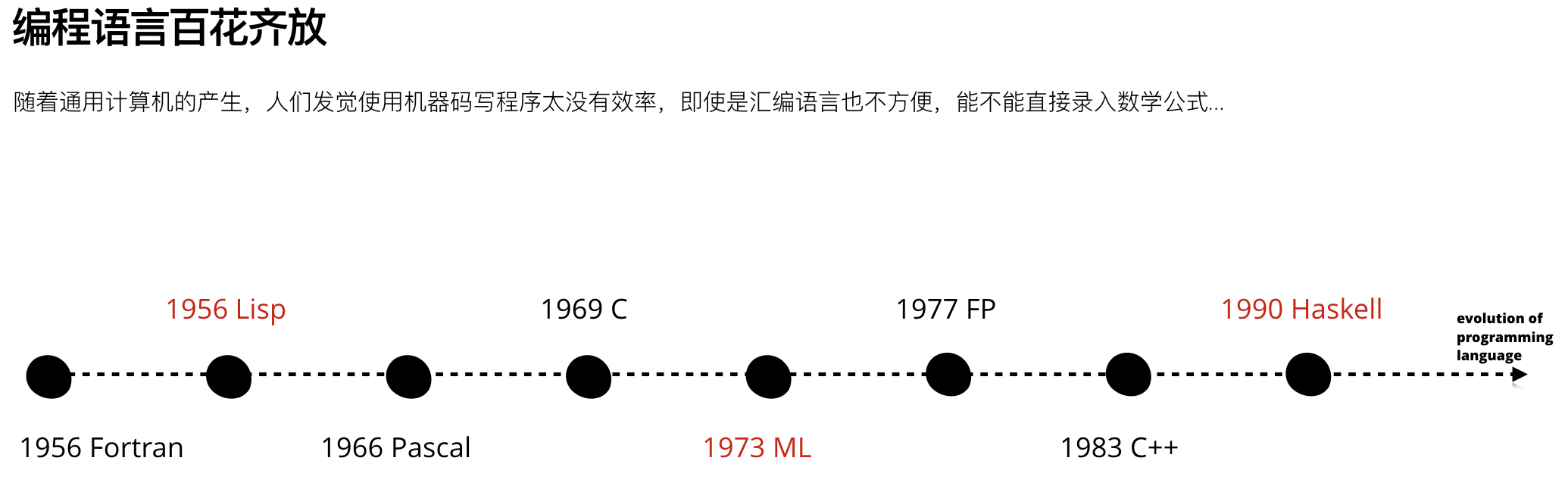### 神奇的 Y Combinator

``````(def Y (fn [f]
((fn [x] (x x))
(fn [x]
(f (fn [y]
((x x) y)))))))
``````

Lisp是动态语言，使用S表达式

ML是第一个使用Hindley-Milner type inference algorithm的语言

## 函数是一等公民

### 1. 作为参数

``````(map inc [1 2 3 4 5]) ;-> (2 3 4 5 6) ;; inc is an argument
``````

### 2. 作为返回值

``````(defn add [num]
(fn [other-num] (+ num other-num))) ;; as return-value

(defn flip [f]  ;; as argument and return-value
(fn [x y]
(f y x)))
``````

### 3. 数据结构

``````(def dictionary {:a "abandon"}) ;; map is also a function, data is code.
(dictionary :a) ;-> "abandon"
(:a dictionary) ;-> "abandon"
``````

### 4. 匿名函数

``````((fn [x] (* x x))
2) ;-> 4

(map
(fn [num] (+ 1 num)) ;; anonymous function
[1 2 3 4 5]) ;-> (2 3 4 5 6)
``````

## 纯函数和不可变性

### 引用透明

``````(inc 1) ; -> 2

(= (inc (inc 1)
(inc 2))) ; -> true
``````

``````(defn fibonacci [number]
(if (or (zero? number) (= 1 number)) 1
(+
(fibonacci (dec number))
(fibonacci (- number 2)))))
(fibonacci 30) ; -> "Elapsed time: 185.690208 msecs"

(def fibonacci
(memoize (fn [number] ;;
(if (or (zero? number) (= 1 number)) 1
(+
(fibonacci (dec number))
(fibonacci (- number 2)))))))
(fibonacci 30) ; -> "Elapsed time: 0.437114 msecs"
``````

### 不可变计算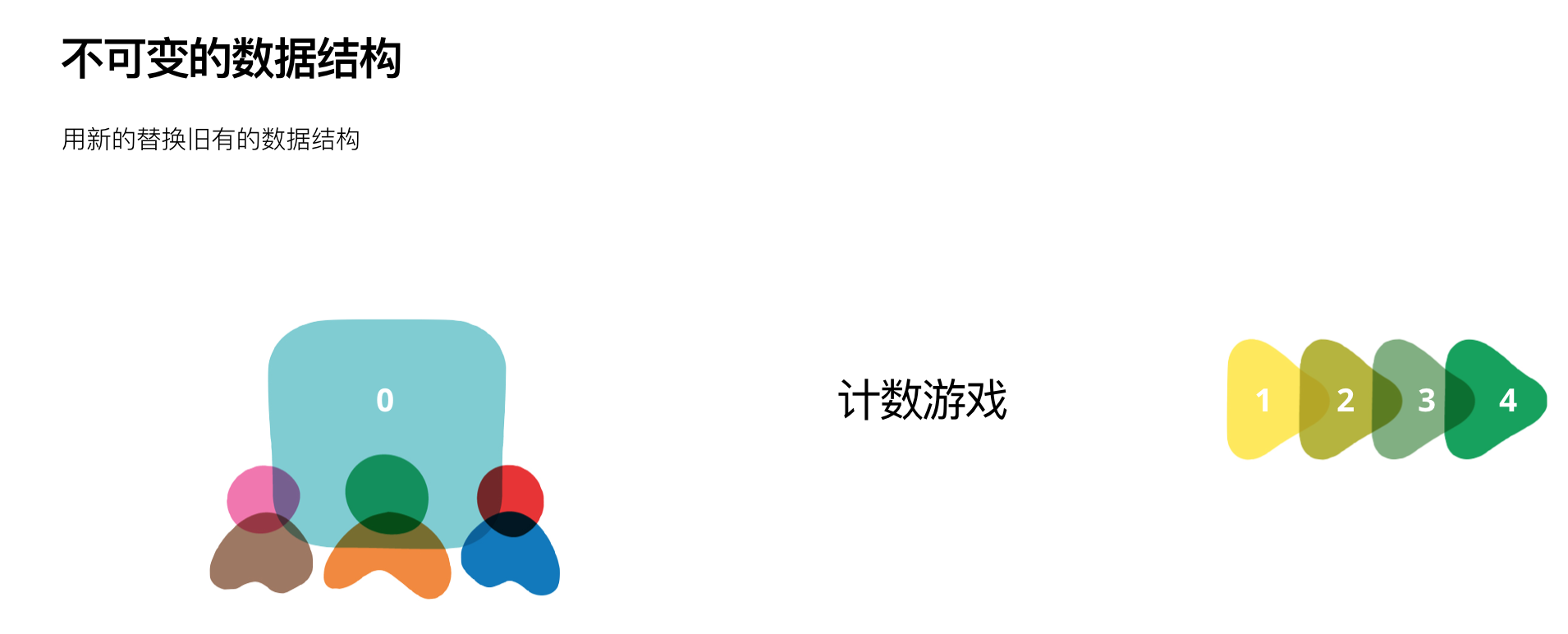### 不可变的链式结构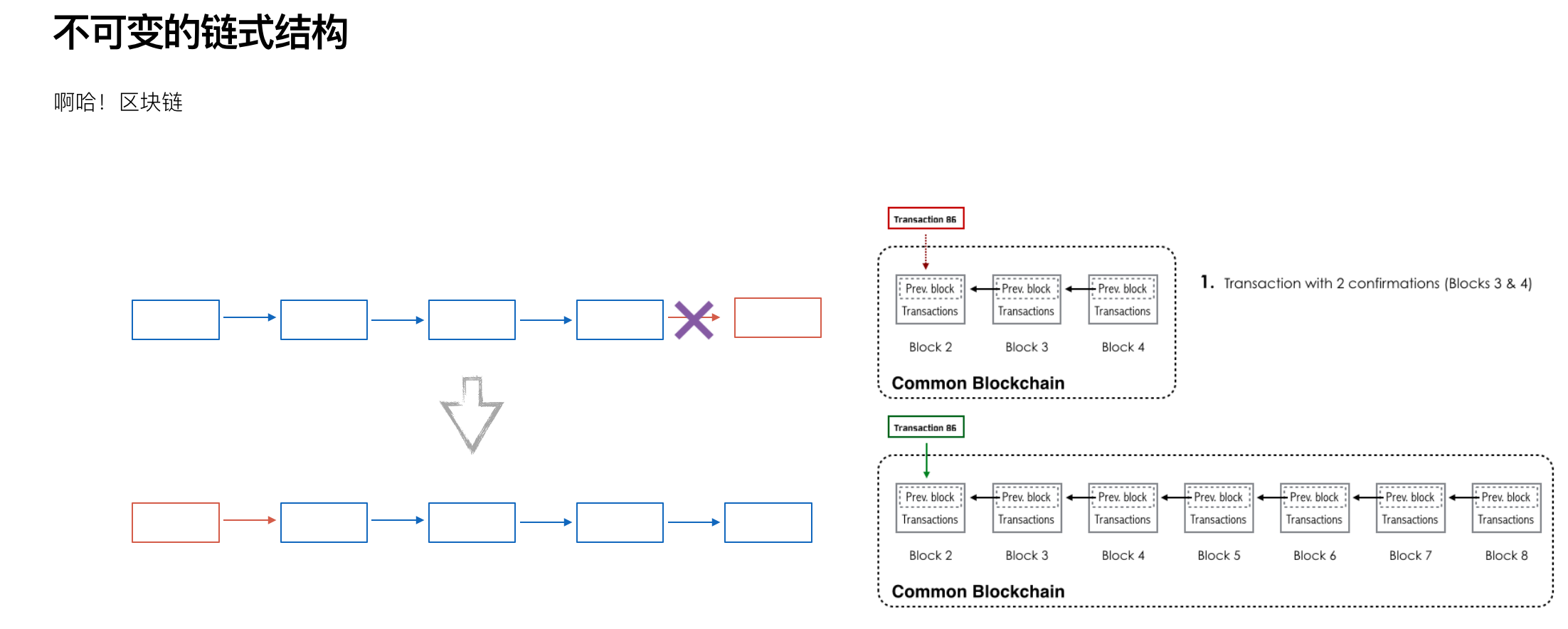Clojure的创造者Rich Hickey扩展了Ideal Hash Tree数据结构，实现了Persistent Vector。由于此处的叶子节点可以扩展成32个，所以可以大量存储数据。利用Ideal Hash Tree的特点可以快速索引出数据，与此同时，数据的“增删改”也能做到近常数化的时间，并且总是产生新的数据结构替换原有的数据结构，即一种不可变的链式存储结构。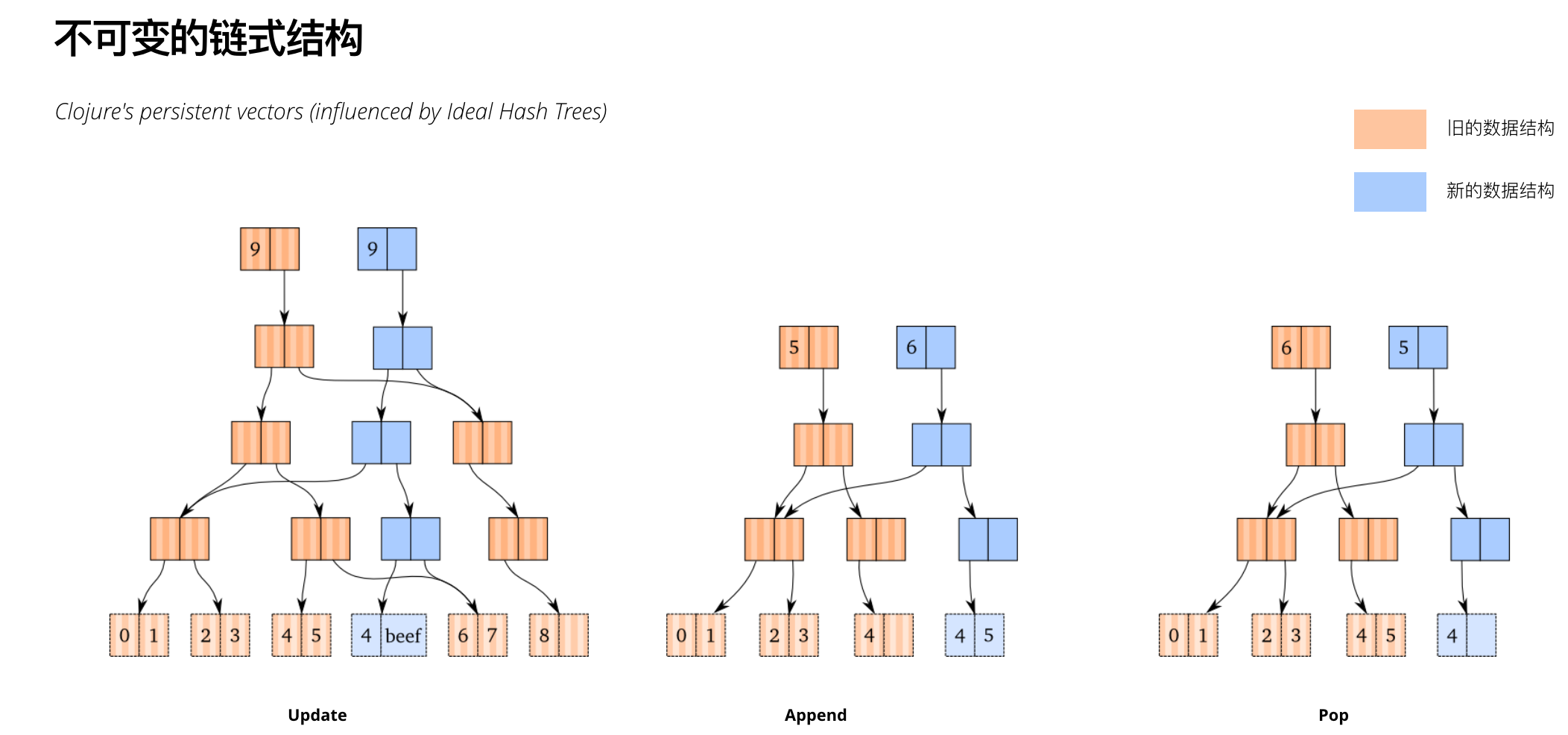### 不可变的树状结构

Zipper数据结构类似于文本编辑器中的 gap buffer，编辑文本时，光标左边和右边分别是独立的buffer，光标处也是单独的buffer，这样便可以方便地添加文字，也很方便删除左右buffer中的文字；移动光标会涉及buffer之间的拷贝。基本上能在常数时间内完成编辑。Zipper数据结构模仿了这种方式，能在常数时间内完成树的编辑工作，也能很快地重新构建一棵树。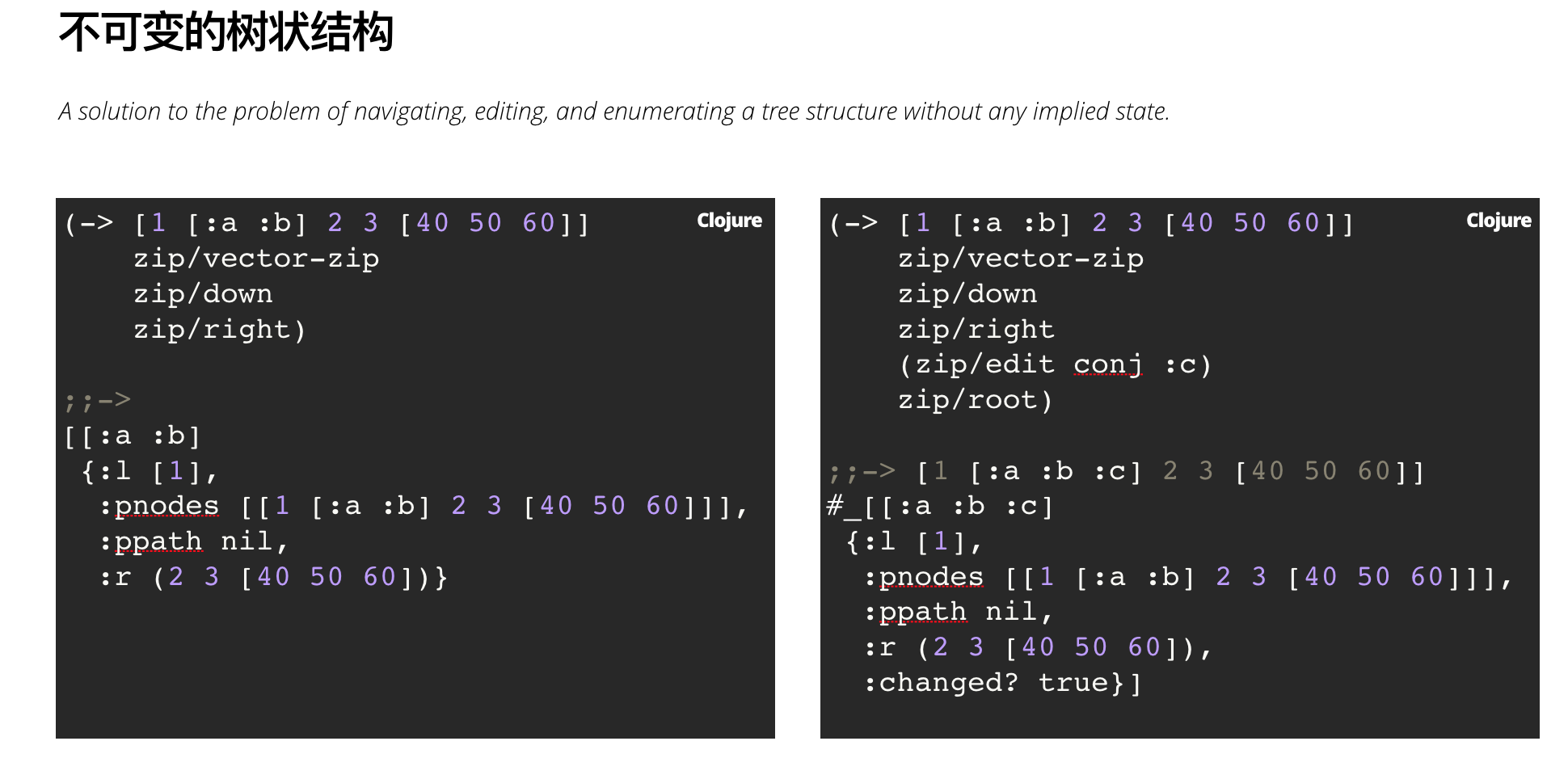## 递归

``````(defn reverse-seq [coll]
(when-let [elem (first coll)]
(concat (reverse-seq (rest coll)) [elem])))
(reverse-seq [1 2 3]) ; -> (3 2 1)
``````

``````(defn gcd [& nums]
(reduce #(if (zero? %2)
%
(recur %2 (mod % %2))) nums))
(gcd 8 16) ; -> 8
``````

## 生成式测试

``````(defn add [a b]
(+ a b))
;; 任取两个整数，把a和b加起来的结果减去a总会得到b。
(prop/for-all [a (gen/int)
b (gen/int)]
(= (- (add a b) a) b)))

; -> {:result true, :num-tests 100, :seed 1515935038284}
``````

## 抽象是什么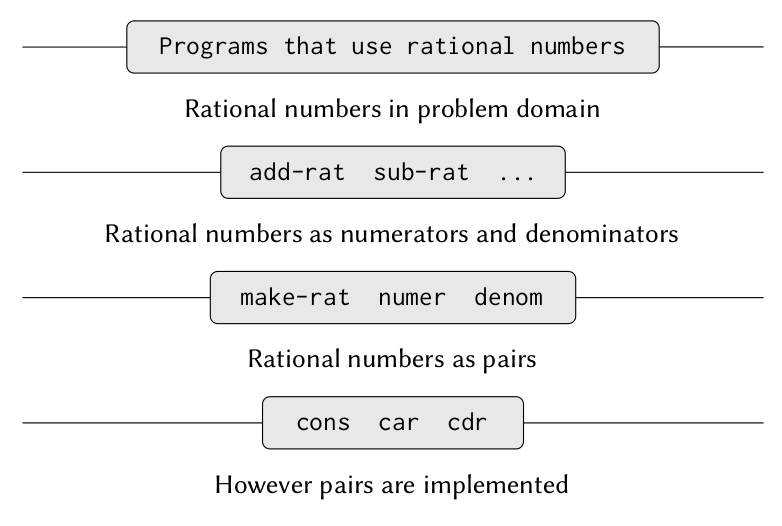### 命名

``````(* 3 3 3)
(* x x x)
(* y y y)
->
(defn cube [x]
(* x x x))
``````

### 延迟决策

``````pair:: (cons x y)
firstpair -> x
secondpair -> y
``````

``````(defn cons [x y]
(fn [m]
(cond (= m 0) x
(= m 1) y)))
(defn first [z]
(z 0))
(defn second [z]
(z 1))
``````

``````(defn cons [x y]
(fn [b]
(b x y))
(defn first [z]
(z (fn [x y] x)))
(defn second [z]
(z (fn [x y] y)))
``````

## 高阶函数

### 过程抽象

``````(map inc [1 2 3 4 5]) ; -> (2 3 4 5 6)
``````

## 函数的组合

### 神奇的加法

``````(((comp (map inc) (filter odd?)) +) 1 2) ; -> 4
``````

``````(def special+ ((comp (map inc) (filter odd?)) +))
(special+ 1 2) ; -> 4

; <=> 等价于
(if (odd? (inc 2))
(+ 1 3))
1)
``````

### transducer

``````(def xf (comp (filter odd?) (take 10)))
(transduce xf conj (range))
;; [1 3 5 7 9 11 13 15 17 19]
``````

``````(->> (range)
(filter odd?)
(take 10))
``````

``````(transduce xf + (range)) ;-> 100
``````

``````(defn log [& [idx]]
(fn [rf]
(fn
([] (rf))
([result] (rf result))
([result el]
(let [n-step (if idx (str "Step: " idx ". ") "")]
(println (format "%sResult: %s, Item: %s" n-step result el)))
(rf result el)))))

(def ^:dynamic *dbg?* false)

(defn comp* [& xforms]
(apply comp
(if *dbg?*
(->> (range)
(map log)
(interleave xforms))
xforms)))

(binding [*dbg?* true]
(transduce
(comp*
(map inc)
(filter odd?))
+
(range 5))) ;; -> 9

Step: 0. Result: 0, Item: 1
Step: 1. Result: 0, Item: 1
Step: 0. Result: 1, Item: 2
Step: 0. Result: 1, Item: 3
Step: 1. Result: 1, Item: 3
Step: 0. Result: 4, Item: 4
Step: 0. Result: 4, Item: 5
Step: 1. Result: 4, Item: 5
``````

``````(defn m [f]
(fn [rf]
(fn [result elem]
(rf result (f elem)))))
``````

``````(comp (m f) (m1 f1) ...)
``````

``````((m f)
((m1 f1)
((m2 f2) ...)))
->
(fn [result elem]
(((m1 f1)
((m2 f2) ...)) result (f elem)))
``````

1. xform 作为组合的前提
2. 执行顺序从左到右；
3. + 作为 reducing function 最后执行；

1. Identity—For a monad m, m flatMap unit => m
2. Unit—For a monad m, unit(v) flatMap f => f(v)
3. Associativity—For a monad m, m flatMap g flatMap h => m flatMap {x => g(x) flatMap h}
``````// java8 实现的 9*9 乘法表
private List<T> elements;

this.elements = singletonList(elem);
}

this.elements = elems;
}

List<U> newElements = new ArrayList<>();
}

public <X> ListMonad<X> uint(X elem) {
}

return m.flatmap(this::map);
}

public <U> ListMonad<U> map(Function<T, U> fn) {
return flatmap(t -> uint(fn.apply(t)));
}

public static void main(String[] args) {
ListMonad<Integer> m = new ListMonad<>(Arrays.asList(1, 2, 3, 4, 5, 6, 7, 8, 9));
ListMonad<Integer> m1 = new ListMonad<>(Arrays.asList(1, 2, 3, 4, 5, 6, 7, 8, 9));

ListMonad<Integer> list = m.apply(m1.map(x -> y -> x * y));
// [1...81]
}
}
``````

## 表达式优于语句

### S表达式

1. 原子，或者；
2. 形式为 (x • y) 的表达式，其中x和y也是S表达式。

``````(-> [1 2 3]
(->> (map inc)
(filter odd?)
(sort)
(first))
(or :not-found))
; -> 3
(-> [1 1 3]
(->> (map inc)
(filter odd?)
(sort)
(first))
(or :not-found)
; -> :not-found
``````

``````(if-let [r (first (sort (filter odd? (map inc [1 1 1]))))]
r
:not-found)
; -> :not-found
``````

### if-else -> Optional

``````Optional<Rule> rule = ruleOf(id);
if(rule.isPresent()) {
return transform(rule.get());
} else {
throw new RuntimeException();
}

public Rule transform(Rule rule){
return Rule.builder()
.withName("No." + rule.getId())
.build();
}
``````

``````Optional rule = ruleOf(id);

if(!rule.isPresent()) {
throw new RuntimeException();
}

return transform(rule.get());
``````

``````...
return rule.map(r -> transform(r)).get();
``````

``````...
rule.map(r -> Rule.builder()
.withName("No." + r.getId())
.build()).get();
``````

``````...
rule.map(r-> Rule.builder()
.withName("No." + r.getId())
.build())
.orElseThrow(() ->new RuntimeException());
``````

``````if(!rule.isPresent()) {
// throw new RuntimeException();
}
``````

``````ruleOf(id).map(r-> Rule.builder()
.withName("No." + r.getId())
.build())
.orElseThrow(() ->new RuntimeException());
``````

## 我们认识事物的方式

1. 把几个简单的想法合并成一个复合概念，从而创造出所有复杂的概念。
2. 简单的或复杂的两种思想融合在一起，并立即把它们联系起来，不要把它们统一起来，从而得到它所有的关系思想。
3. 把他们与其他所有陪伴他们的真实存在的想法分开：这就是所谓的抽象，因此所有的一般想法都是被提出来的。